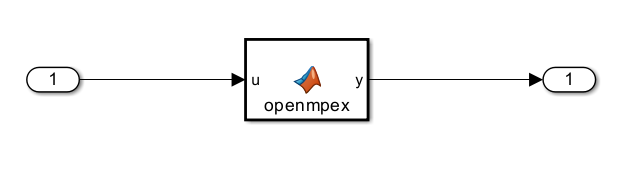## Generate Parallel `for`-Loops Using the Open Multiprocessing (OpenMP) Application Interface

When you generate code from MATLAB Function, MATLAB System, and For Each subsystem blocks, by default the code generator produces code that implements `for`-loops in a single thread. If you have Parallel Computing Toolbox™, you can specify that these `for`-loops run in parallel by selecting the Generate parallel for-loops parameter and by including the `parfor` statement in your MATLAB Function block and MATLAB System block code. Running iterations in parallel can significantly improve the execution speed of the generated code.

The code generator implements the `for`-loops in parallel by using the Open Multiprocessing (OpenMP) application interface to support shared-memory, multicore code generation. By default, the code generator uses as many threads as it finds available. For MATLAB Function and MATLAB System blocks using `parfor`, if you specify the number of threads to use, the code generator uses at most that number of threads, even if additional threads are available. The code generator cannot generate parallel `for`-loops for For Each Subsystem blocks that contain S-functions.

### Generate OpenMP Code from MATLAB Function or MATLAB System Blocks

In this example, you generate OpenMP code from a MATLAB Function block that contains the `parfor` statement as part of its code. Use the `parfor` to speed up execution when you have many iterations of a simple calculation or a loop iteration takes a long time to execute. For more on how `parfor`-loops improve execution speed and when to use them, see Algorithm Acceleration Using Parallel for-Loops (parfor).

1. Create a model that contains a MATLAB Function or MATLAB System block. This example uses a MATLAB Function block.

2. Add this code to the MATLAB Function block.

```function y = openmpex(u) %#codegen % Copyright 2019 The MathWorks, Inc. A = ones(20,50); t = 0; parfor (i = 1:10,4) A(i,1) = A(i,1) + 1; end y = A(1,4) + u + t;```

Note the `parfor` input value of `4` specifies the maximum number of threads to use.

3. Connect the blocks.4. Open the Configuration Parameters dialog box. On the Code Generation pane, and set the System Target File parameter to `ert.tlc`.

5. On the Solver pane, set the Type parameter to `Fixed-step`.

6. On the Optimization pane, set the Priority parameter to ```Maximize execution speed```. Now, the Generate parallel for-loops parameter is automatically selected. This parameter enables the compiler to compute loops in parallel.

7. Build the model and generate code.

In the generated code, the pragma instructs the compiler to execute the looping in OpenMP parallel `for`-loops through multithreading:

```#pragma omp parallel for num_threads(4 > omp_get_max_threads() ? omp_get_max_threads() : 4)```

The number `4` indicates the number of processing threads.

Because the loop body can execute in parallel on multiple threads, it must conform to certain restrictions. If Embedded Coder™ detects loops that do not conform to `parfor` specifications, it produces an error. For more information, see parfor Restrictions.